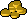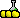Infobox Recipe
Value
Unknown edit-
Unknown edit Unknown edit Unknown edit -
Requirements
Requirements unknown edit
Materials
Materials unknown edit
Template documentation [purge]
This documentation is transcluded from Template:Infobox Recipe/doc.

The Recipe template is used to show the details of a recipe in item articles.

### Usage

```{{Infobox Recipe
|name        = <!-- Name of the produced item. -->
|image       = <!-- Image of the item. -->
|skill       = <!-- The skill this recipe belongs to. If not related to a skill, put "no". -->
|skillXP     = <!-- The XP given to the main skill. -->
|storeValue  = <!-- The store sell value of the product. -->
|alchValue   = <!-- The high-alchemy of the product. -->
|secSkill    = <!-- The secondary skill this recipe belongs to. If none, leave empty-->
|secXP       = <!-- XP given in the secondary skill. -->

|level       = <!-- The level required in the main skill of the recipe. Leave empty if not related to a skill. -->
|quest       = <!-- Quest requirement of the recipe. -->

|mat1        = <!-- The name of the first material. -->
|mat1qty     = <!-- The quantity of the first material. -->
|mat1img     = <!-- The inventory image of the first material. -->
|mat2        = <!-- The name of the second material. Leave empty if none. -->
|mat2qty     = <!-- The quantity of the second material. Leave empty if none. -->
|mat2img     = <!-- The inventory image of the second material. Leave empty if none. -->
|mat3        = <!-- The name of the third material. Leave empty if none. -->
|mat3qty     = <!-- The quantity of the third material. Leave empty if none. -->
|mat3img     = <!-- The inventory image of the third material. Leave empty if none. -->
|mat4        = <!-- The name of the fourth material. Leave empty if none. -->
|mat4qty     = <!-- The quantity of the fourth material. Leave empty if none. -->
|mat4img     = <!-- The inventory image of the fourth material. Leave empty if none. -->
|mat5        = <!-- The name of the fifth material. Leave empty if none. -->
|mat5qty     = <!-- The quantity of the fifth material. Leave empty if none. -->
|mat5img     = <!-- The inventory image of the fifth material. Leave empty if none. -->
|mat6        = <!-- The name of the sixth material. Leave empty if none. -->
|mat6qty     = <!-- The quantity of the sixth material. Leave empty if none. -->
|mat6img     = <!-- The inventory image of the sixth material. Leave empty if none. -->
}}
```

### Example

```{{Infobox Recipe
|name = Prayer potion (3)
|image=[[File:Prayer potion(3).png]]
|skill = Herblore
|skillXP = 30.5
|storeValue = 4000
|alchValue = 4000
|level = 40
|mat1=Ranarr potion (unf)
|mat1img=[[File:Ranarr potion (unf).png]]
|mat1qty=1
|mat2=Snape grass
|mat2img=[[File:Snape_grass.png]]
|mat2qty=1
}}
```

### Blank template

```{{Infobox Recipe
|name       =
|image      =
|skill      =
|skillXP    =
|storeValue =
|alchValue  =
|level     =
|questReq  =
|mat1      =
|mat1img   =
|mat1qty   =
|mat2      =
|mat2img   =
|mat2qty   =
|mat3      =
|mat3img   =
|mat3qty   =
|mat4      =
|mat4img   =
|mat4qty   =
|mat4price =
|mat5      =
|mat5qty   =
|mat6      =
|mat6img   =
|mat6qty   =
}}
```